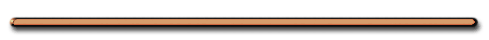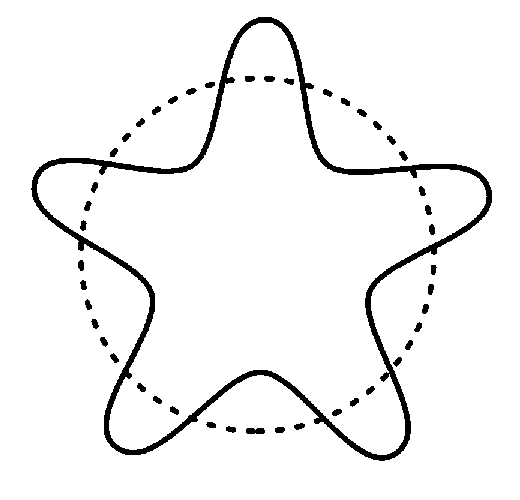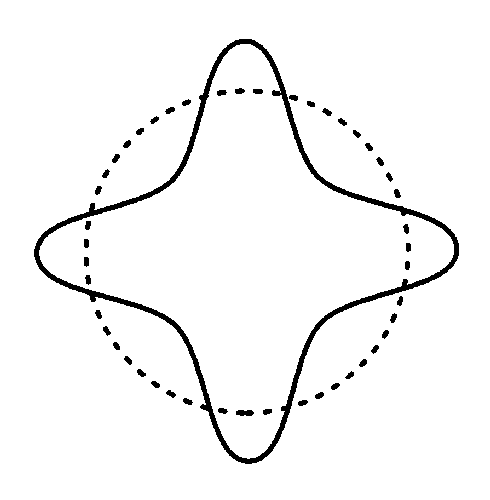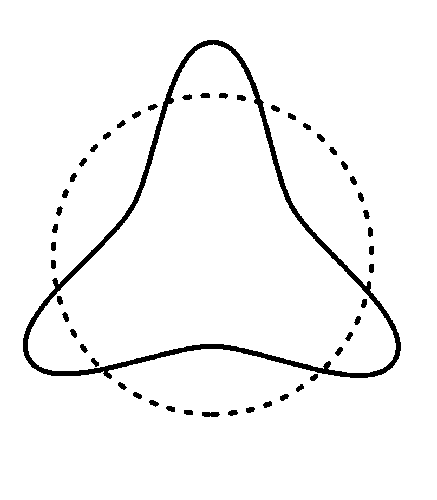HomeWhy were electrons limited
in the energies they can possess?

Electron Standing Waves

School blocks YouTube? Use the file below.

St. Mary's U. Astronomy and Physics Dept.

1. An electron is a standing wave. (A bound wave)5 wavelengths4 wavelengths3 wavelengths2 wavelengths

2. Circular standing waves can only be a whole number of wavelengths3. Limited wavelengths mean limited possible energies

Possible Electron Circumferences

= 2∏r = nλ

where n = 1,2,3,4 etc

Wavelength - describes probability of finding an electron at particular position.

Electron is a wave circled in on itself

This standing wave has four cyclesThe angular (circular) momentum of
an electron is limited ...

...because a circular electron wave must be a whole number of wavelengths

Then C = 2(∏)r = nλ

where n = 1,2,3,4 etc

And λ = h/p = h/mv

Then … 2()r = nh/mv

Angular Momentum

=

mvr  = n[h/2∏]

 Angular momentum (mvr) is restricted to whole number multiples  of h/2∏Limitations